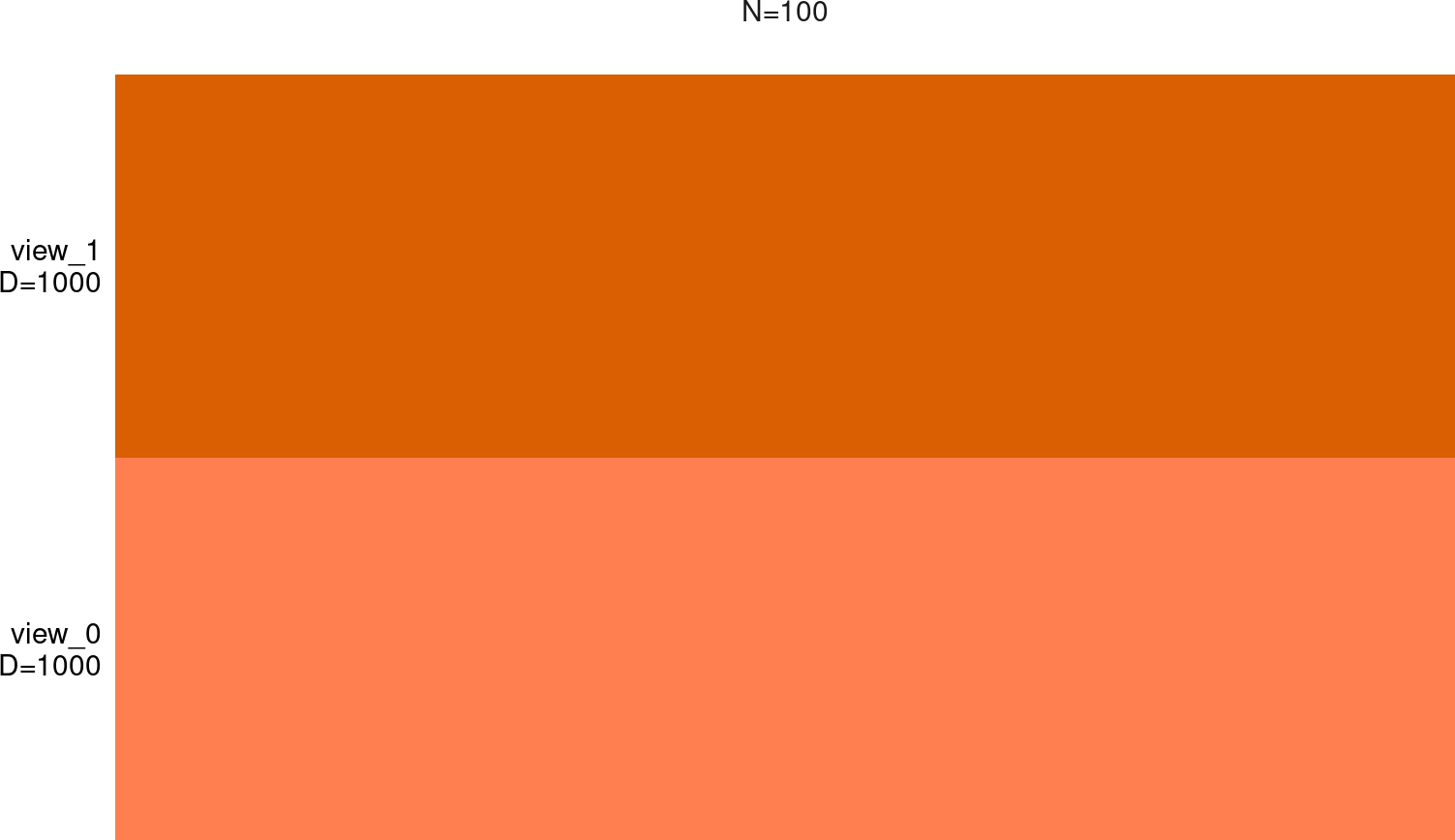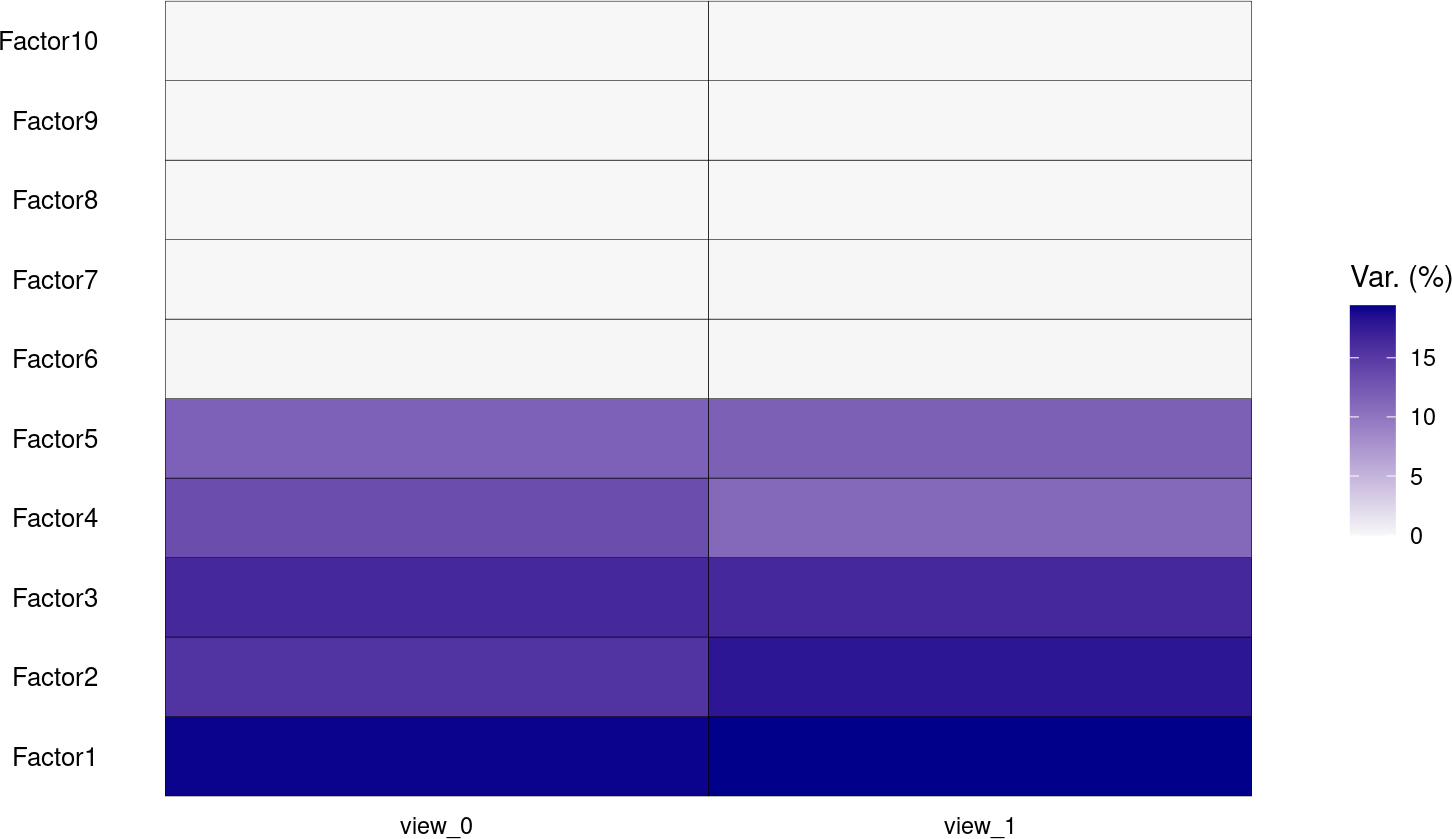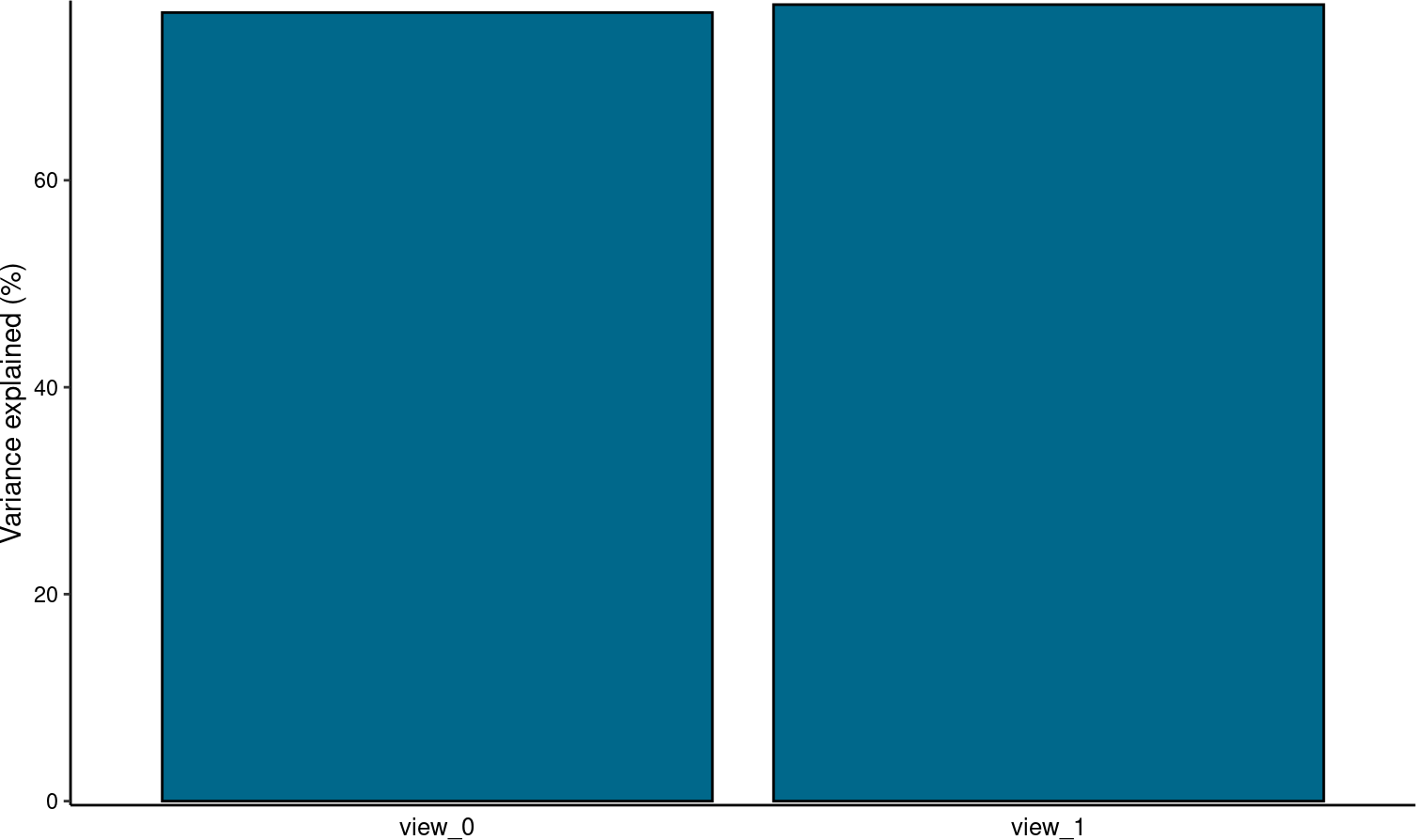# 1 Introduction

In the MOFA2 R package we provide a wide range of downstream analysis to visualise and interpret the model output. Here we provide a brief description of the main functionalities. This vignette is made of simulated data and we do not highlight biologically relevant results. Please see our tutorials for real use cases.

``````library(ggplot2)
library(MOFA2)``````

``````filepath <- system.file("extdata", "model.hdf5", package = "MOFA2")

## 3.1 Overview of data

The function `plot_data_overview` can be used to obtain an overview of the input data. It shows how many views (rows) and how many groups (columns) exist, what are their corresponding dimensionalities and how many missing information they have (grey bars).

``plot_data_overview(model)``The metadata is stored as a data.frame object in `model@samples_metadata`, and it requires at least the column `sample`. The column `group` is required only if you are doing multi-group inference.
The number of rows must match the total number of samples in the model (`sum(model@dimensions\$N)`).

``````Nsamples = sum(get_dimensions(model)[["N"]])

sample = samples_names(model)[],
condition = sample(c("A","B"), size = Nsamples, replace = TRUE),
age = sample(1:100, size = Nsamples, replace = TRUE)
)

``````##             sample condition age        group
## 1 sample_0_group_1         B  31 single_group
## 2 sample_1_group_1         B  70 single_group
## 3 sample_2_group_1         A  79 single_group``````

# 5 Variance decomposition

The first step in the MOFA analysis is to quantify the amount of variance explained (\(R^2\)) by each factor in each data modality.
The variance explained estimates are stored in the hdf5 file and loaded in `model@cache[["variance_explained"]]`:

``````# Total variance explained per view
``````##   view_0   view_1
## 76.20973 76.97777``````
``````# Variance explained for every factor in per view
``````##              view_0      view_1
## Factor1 19.20399955 19.41070871
## Factor2 15.47560732 17.94710458
## Factor3 16.47469843 16.48996544
## Factor4 13.42094721 11.09844071
## Factor5 11.76334520 11.82572116
## Factor6  0.03885712  0.07087386``````

Variance explained estimates can be plotted using `plot_variance_explained(model, ...)`. Options:

• factors: character vector with a factor name(s), or numeric vector with the index(es) of the factor(s). Default is “all”.
• x: character specifying the dimension for the x-axis (“view”, “factor”, or “group”).
• y: character specifying the dimension for the y-axis (“view”, “factor”, or “group”).
• split_by: character specifying the dimension to be faceted (“view”, “factor”, or “group”).
• plot_total: logical value to indicate if to plot the total variance explained (for the variable in the x-axis)

In this case we have 5 active factors that explain a large amount of variation in both data modalities.

``plot_variance_explained(model, x="view", y="factor")``The model explains ~70% of the variance in both data modalities.

``plot_variance_explained(model, x="view", y="factor", plot_total = TRUE)[]``# 6 Visualisation of Factors

The MOFA factors capture the global sources of variability in the data. Mathematically, each factor ordinates cells along a one-dimensional axis centered at zero. The value per se is not important, only the relative positioning of samples matters. Samples with different signs manifest opposite “effects” along the inferred axis of variation, with higher absolute value indicating a stronger effect. Note that the interpretation of factors is analogous to the interpretation of the principal components in PCA.

## 6.1 Visualisation of factors one at a time

Factors can be plotted using `plot_factor` (for beeswarm plots of individual factors) or `plot_factors` (for scatter plots of factor combinations).

``````plot_factor(model,
factor = 1:3,
color_by = "age",
shape_by = "condition"
)``````